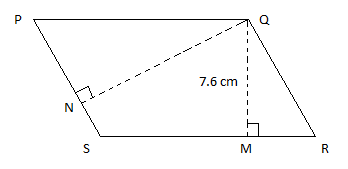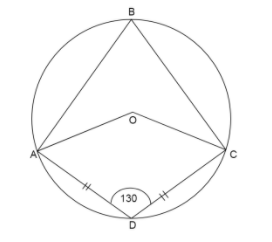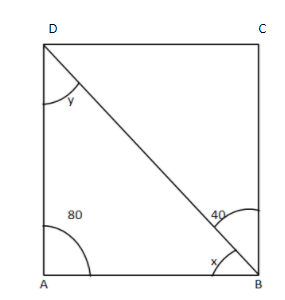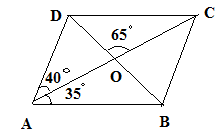Filters
Latest Questions
CBSE
Mathematics
Angles of a parallelogram
In a parallelogram $ABCD$ if angle $D = 125^\circ$, then the other angles are?
CBSE
Mathematics
Angles of a parallelogram
Show that the angle bisectors of a parallelogram form a rectangle.

CBSE
Mathematics
Angles of a parallelogram
In quadrilateral ABCD,$AB\parallel CD$$\angle D = 2\angle B$, $AD = b$ and $CD = a$ then length of side AB is
A.$a + 4b$
B.$a - 3b$
C.$a - 2b$
D.$a + b$
CBSE
Mathematics
Angles of a parallelogram
Adjacent angles in a parallelogram are
(A) complementary
(B) Supplementary
(C) ${120^ \circ }$
(D) None of the above.

CBSE
Mathematics
Angles of a parallelogram
PQRS is a parallelogram. QM is the height from Q to SR and QN is the height from Q to PS. If SR = 12 cm and QM = 7.6 cm, then find QN if PS = 8 cm.CBSE
Mathematics
Angles of a parallelogram
ABCD is a quadrilateral inscribed in a circle with center O, $\angle ADC = {130^ \circ }$ and $AD = DC$. Calculate:
(i) reflex $\angle AOC$
(ii) $\angle ABC$
(iii) $\angle AOD$CBSE
Mathematics
Angles of a parallelogram
In the given figure $ABCD$ is a parallelogram. Find the angles $x{\text{ and }}y$CBSE
Mathematics
Angles of a parallelogram
In the adjoining figure $ABCD$ is a parallelogram in which $\angle CAD = 40^\circ$, $\angle BAC = 35^\circ$ and $\angle COD = 65^\circ$. Calculate
A) $\angle ABD$
B) $\angle BDC$
C) $\angle CBD$CBSE
Mathematics
Angles of a parallelogram
Is quadrilateral ABCD a parallelogram, if $\angle A=70{}^\circ$ and $\angle C=65{}^\circ$ ? Give reason.
CBSE
Mathematics
Angles of a parallelogram
Find the measure of all the angles of the parallelogram if one angle is 24 less than twice the smallest angle.
CBSE
Mathematics
Angles of a parallelogram
In a parallelogram, the sum of adjacent angles is
A) $90^\circ$
B) $180^\circ$
C) $270^\circ$
D) $360^\circ$
CBSE
Mathematics
Angles of a parallelogram
Sum of the adjacent angles of a parallelogram is equal to
A) 60$^\circ$
B) 90$^\circ$
C) 150$^\circ$
D) 180$^\circ$
Prev
1
2